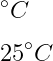# How to denote degree celsius(°C) symbol in LaTeX?

You are all familiar with the degree celsius(°C) symbol. and this symbol is represented by placing degree(°) symbol and C side by side.

In latex, there is no default predefined command to represent the degree celsius(°C) symbol. However, you can define this symbol by using C with the degree(°) symbol. For example …

\documentclass{article}
\begin{document}
$^\circ C$
$25^\circ C$
\end{document}

Output :## Use textcomp and mathcomp package for degree celsius symbol

The textcomp and mathcomp packages contain predefined \textcelsius and \tccentigrad commands to denote the degree celsius symbol. And you can also create this symbol in a textcomp package using C with \textdegree command.

\documentclass{article}
\usepackage{textcomp,mathcomp}
\begin{document}
51 $\tccentigrade$
25 \textdegree C\\
49 \textcelsius
\end{document}

Output :## Use gensymb package for degree celsius symbol

There is no predefined command in this package. So, you need to create a degree celsius symbol using C with the degree symbol.

\documentclass{article}
\usepackage{gensymb}
\begin{document}
$\degree C$
$25 \degree C$
\end{document}

Output :## Use siunitx package for degree celsius symbol

The syntax for this \SI command is slightly different, and temperature value and \celsius or \degreeCelsius commands are used as arguments.

\documentclass{article}
\usepackage{siunitx}
\begin{document}
$\SI{49}{\celsius}$
$\SI{25}{\degreeCelsius}$
\end{document}

Output :#### Md Jidan Mondal

LaTeX expert with over 10 years of experience in document preparation and typesetting. Specializes in creating professional documents, reports, and presentations using LaTeX.